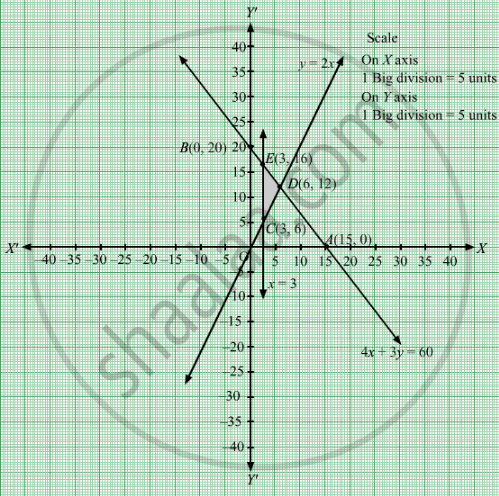# A Box Manufacturer Makes Large and Small Boxes from a Large Piece of Cardboard. the Large Boxes Require 4 Sq. - Mathematics

Sum

A box manufacturer makes large and small boxes from a large piece of cardboard. The large boxes require 4 sq. metre per box while the small boxes require 3 sq. metre per box. The manufacturer is required to make at least three large boxes and at least twice as many small boxes as large boxes. If 60 sq. metre of cardboard is in stock, and if the profits on the large and small boxes are Rs 3 and Rs 2 per box, how many of each should be made in order to maximize the total profit?

#### Solution

Let large boxes and small boxes be manufactured.
Number of boxes cannot be negative.
Therefore, $x \geq 0, y \geq 0$
The large boxes require 4 sq. metre per box while the small boxes require 3 sq. metre per box and if 60 sq. metre of cardboard is in stock.

$4x + 3y \leq 60$
The manufacturer is required to make at least three large boxes and at least twice as many small boxes as large boxes.

$x \geq 3$

$y \geq 2x$

If the profits on the large and small boxes are Rs 3 and Rs 2 per box. Therefore, profit gained by him on large boxes and small boxes is Rs 3x and Rs 2y respectively.

Total profit  = Z = $3x + 2y$

The mathematical formulation of the given problem is

Max Z = $3x + 2y$

subject to

$4x + 3y \leq 60$
$x \geq 3$
$y \geq 2x$

$x \geq 0, y \geq 0$

First we will convert inequations into equations as follows:
4x + 3y = 60, x = 3, y = 2xx = 0 and y = 0
Region represented by 4x + 3y ≤ 60:
The line 4x + 3y = 60 meets the coordinate axes at A(15, 0) and B(0, 20) respectively. By joining these points we obtain the line
4x + 3y = 60. Clearly (0,0) satisfies the 4x + 3y = 60. So, the region which contains the origin represents the solution set of the inequation 4x + 3y ≤ 60.

Region represented by x ​ ≥ 3:
The line ​x = 3 is the line passes through (3, 0) and is parallel to Y axis. The region to the right of the line = 3 will satisfy the inequation
x ​ ≥ 3.

Region represented by y ​ ≥ 2x:
The line ​y = 2is the line that passes through (0, 0). The region above the line y = 2x will satisfy the inequation y ​ ≥ 2x. Like if we take an example taking a point (5, 1) below the line y = 2x .Here, 1 < 10 which does not satisfies the inequation y ​ ≥ 2x. Hence, the region above the line y = 2x will satisfy the inequation y ​ ≥ 2x.

Region represented by ≥ 0 and y ≥ 0:
Since, every point in the first quadrant satisfies these inequations. So, the first quadrant is the region represented by the inequations x ≥ 0, and y ≥ 0.

The feasible region determined by the system of constraints 4x + 3y ≤ 60, x ​ ≥ 3, y ​ ≥ 2xx ≥ 0 and y ≥ 0 are as followsThe corner points are  $\left( 3, 16 \right)$, D(6, 12) and C(3, 6).The values of Z at the corner points are

 Corner points Z =  $3x + 2y$ E 41 D 42 C 21

The maximum value of Z is 42 which is at D
$\left( 6, 12 \right)$ Thus, for maximum profit is Rs 42, 6 units of large boxes and 12 units of smaller boxes should be manufactured.

Concept: Graphical Method of Solving Linear Programming Problems
Is there an error in this question or solution?

#### APPEARS IN

RD Sharma Class 12 Maths
Chapter 30 Linear programming
Exercise 30.4 | Q 34 | Page 54